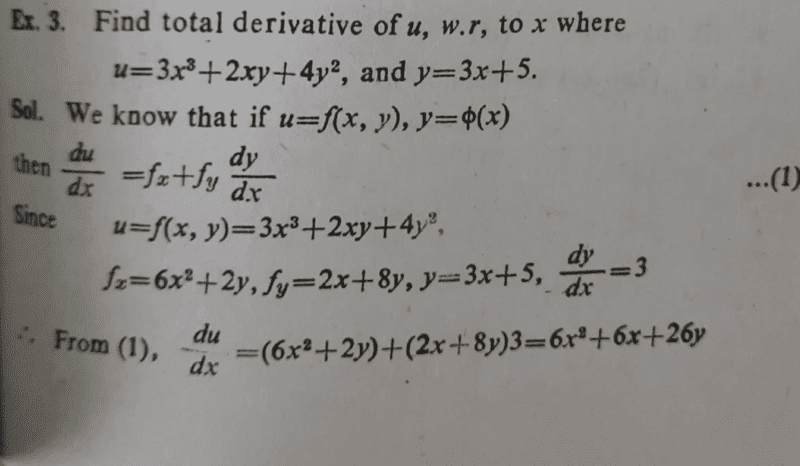# Find the total derivative of ##u## with respect to ##x##

• chwala

#### chwala

Gold Member
Homework Statement
see attached
Relevant Equations
total derivatives
see attached below; the textbook i have has many errors...clearly ##f_x## is wrong messing up the whole working to solution...we ought to have;
##\frac {du}{dx}=(9x^2+2y)+(2x+8y)3=9x^2+2y+6x+24y=9x^2+6x+26y##

Last edited:
$$\frac{du}{dx}=9x^2+2y+2x\frac{dy}{dx}+8y\frac{dy}{dx}=9x^2+2(3x+5)+2x3+8(3x+5)3$$
Expression ##u_x## seems ambiguous to me. How can we change x without changing y?

Last edited:
•chwala
chwala said:
Homework Statement:: see attached
Relevant Equations:: total derivatives

see attached below; the textbook i have has many errors...

View attachment 296761

clearly ##f_x## is wrong messing up the whole working to solution...we ought to have;
##u_x=(9x^2+2y)+(2x+8y)3=9x^2+2y+6x+24y=9x^2+6x+26y##
Their answer has a typo. The very first term on the right side should be ##9x^2##, not ##6x^2##.

•chwala
anuttarasammyak said:
$$\frac{du}{dx}=9x^2+2y+2x\frac{dy}{dx}+8y\frac{dy}{dx}=9x^2+2(3x+5)+2x3+8(3x+5)3$$
Expression ##u_x## seems ambiguous to me. How can we change x without changing y?
yeah..let me amend that... we want total derivative and not partial derivative...i posted this using my android phone and looks like i did not get it right...•anuttarasammyak